Electron. J. Diff. Eqns., Vol. 2006(2006), No. 102, pp. 1-9.

### Existence of positive solutions for higher order singular sublinear elliptic equations Imed Bachar

Abstract:
We present existence result for the polyharmonic nonlinear problem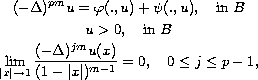in the sense of distributions. Here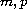are positive integers,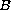is the unit ball in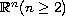and the nonlinearity is a sum of a singular and sublinear terms satisfying some appropriate conditions related to a polyharmonic Kato class of functions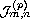.

Submitted May 10, 2006. Published August 31, 2006.
Math Subject Classifications: 34B27, 35J40.
Key Words: Green function; higher-order elliptic equations; positive solution; Schauder fixed point theorem.

Show me the PDF file (238K), TEX file, and other files for this article.Imed Bachar Département de mathématiques Faculté des sciences de Tunis campus universitaire, 2092 Tunis, Tunisia email: Imed.Bachar@ipeit.rnu.tn